Courses

# Triangles, NCERT Solutions, Class 9, Maths Class 9 Notes | EduRev

## Class 9 : Triangles, NCERT Solutions, Class 9, Maths Class 9 Notes | EduRev

The document Triangles, NCERT Solutions, Class 9, Maths Class 9 Notes | EduRev is a part of Class 9 category.
All you need of Class 9 at this link: Class 9

### Theorems with logical proofs

Theorem 1. : If a ray stands on a line, then the sum of the adjacent angles so formed is 180°.
Given: The ray PQ stands on the line XY.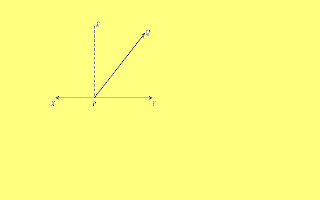To Prove: m∠QPX + m∠YPQ = 180°.
Construction: Draw PE perpendicular to XY.
Proof: m∠QPX = m∠QPE + m∠EPX
m∠QPE + 90° (1)
m∠YPQ = m∠YPE − m∠QPE
90° − m∠QPE (2)
(1) + (2) ⇒ m∠QPX + m∠YPQ = (m∠QPE + 90°) + (90° − m ∠QPE) = 180°.
Thus the theorem is proved.

Theorem 2: If two lines intersect, then the vertically opposite angles are of equal measure.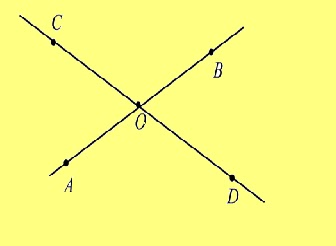Given: Two lines AB and CD intersect at the point O
To prove: m∠AOC = m∠BOD, m∠BOC = m∠AOD.
Proof: The ray OB stands on the line CD.

∴ m∠BOD + m∠BOC = 180° (1)
The ray OC stands on the line AB.
∴ m∠BOC + m∠AOC = 180° (2)
From (1) and (2),
m∠BOD + m∠BOC = m∠BOC + m∠AOC
∴ m∠BOD = m∠AOC.
Since the ray OA stands on the line CD,
m∠AOC + m∠AOD = 180° (3)
From (2) and (3), we get
m∠BOC + m∠AOC = m∠AOC + m∠AOD
∴ m∠BOC = m∠AOD.
Hence the theorem is proved.

Theorem 3: The sum of the three angles of a triangle is 180°.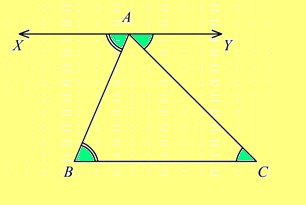Given: ABC is a triangle (see Figure 6.54).
To prove: + ∠+ ∠= 180°.
Construction: Through the vertex A, draw the line
XY parallel to the side BC.
Proof: XY || BC ∴ m∠XAB = m∠ABC (alternate angles).
= ∠B. (1)
Next, AC is a transversal to the parallel lines XY and BC.
∴ m∠YAC = m∠ACB (alternate angles) = ∠C. (2)
We also have m∠BAC = m∠A. (3)
(1) + (2) + (3) ⇒ m∠XAB + m∠YAC + m∠BAC = m∠+ m∠+ m∠A
⇒ (m∠XAB + m∠BAC) + m∠CAY = m∠+ m∠+ m∠C
⇒ m∠XAC + m∠CAY = m∠+ m∠+ m∠C
⇒ 180° = m∠+ m∠+ m∠C.
Hence the theorem is proved.

Theorem4. : The angles opposite to equal sides of a triangle are equal.
Given: ABC is a triangle where AB AC).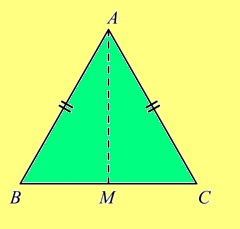To prove: = ∠C.
Construction: Mark the mid point of BC as and join AM.
Proof: In the triangles AMB and AMC
(i) BM CM (ii) AB AC (iii) AM is common.
∴ By the SSS criterion, ΔAMB ≡ ΔAMC.
∴ Corresponding angles are equal. In particular, ∠= ∠C.
Hence the theorem is proved.

Theorem 5. : The side opposite to the larger of two angles in a triangle is longer than the side opposite to the smaller angle.
Given: ABC is a triangle, where ∠is larger than ∠C, that is m∠> m∠C.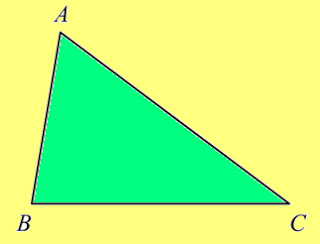To prove: The length of the side AC is longer than the length of the side AB.
i.e., AC AB (see Figure 6.56).
Proof: The lengths of AB and AC are positive numbers. So three cases arise
(i) AC AB
(ii) AC AB
(iii) AC AB
Case (i) Suppose that AC AB. Then the side AB has longer length than the side AC. So the angle ∠which is opposite to AB is larger measure than that of ∠which is opposite to the shorter side AC. That is, m∠> m∠B. This contradicts the given fact that m∠> m∠C. Hence the assumption that AC AB is wrong.
AC AB.
Case (ii) Suppose that AC AB. Then the two sides AB and AC are equal. So the angles opposite to these sides are equal. That is ∠= ∠C. This is again a contradiction to the given fact that ∠> ∠C. Hence AC AB is impossible. Now Case (iii) remains alone to be true.
Hence the theorem is proved.

Theorem 6. : A parallelogram is a rhombus if its diagonals are perpendicular.
Given: ABCD is a parallelogram where the diagonals AC and BD are perpendicular.
To prove: ABCD is a rhombus.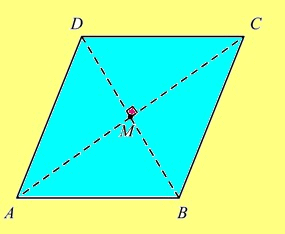Construction: Draw the diagonals ACand BD. Let be the point of intersection of AC and BD (see Proof: In triangles AMB andBMC,
(i) ∠AMB = ∠BMC = 90°
(ii) AM MC
(iii) BM is common.
∴ By SSA criterion, ΔAMB ≡ ΔBMC.
∴Corresponding sides are equal.
In particular, AB BC.
Since ABCD is a parallelogram, AB CDBC AD.
Hence ABCD is a rhombus. The theorem is proved.

Theorem 7:Prove that the bisector of the vertex angle of an isosceles triangle is a median to the base.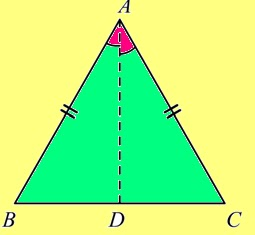Solution: Let ABC be an isosceles triangle where AB AC. Let AD be the bisector of the vertex angle ∠A. We have to prove thatAD is the median of the base BC. That is, we have to prove that is the mid point of BC. In the triangles ADB and ADC,
∴ By SAS criterion, ∠ABD ≡ ΔACD.
∴ The corresponding sides are equal.
∴ BD DC.
i.e., is the mid point of BC.

Theorem 8 Prove that the sum of the four angles of a quadrilateral is 360°.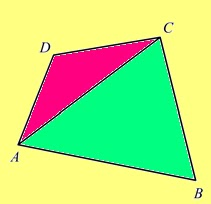Solution: Let ABCD be the given quadrilateral. We have to prove that ∠+ ∠+ ∠+ ∠= 360°. Draw the diagonal AC. From the trianglesACD and ABC, we get
DAC + ∠+ ∠ACD = 180° (1)
CAB + ∠+ ∠ACB = 180° (2)
(1) (2) ⇒ ∠DAC + ∠+ ∠ACD + ∠CAB
+ ∠+ ∠ACB = 360°

⇒ (∠DAC + ∠CAB) + ∠+ (∠ACD + ∠ACB)+ ∠= 360°
⇒ ∠+ ∠+ ∠+ ∠= 360°.

Theorem 8: In a rhombus, prove that the diagonals bisect each other at right angles.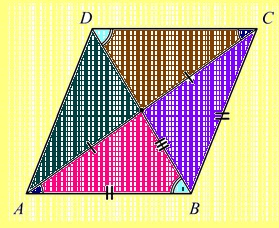Solution: Let ABCD be a rhombus, Draw the diagonals ACand BD. Let them meet at O. We have to prove that is the mid point of both AC and BD and that AC is perpendicular (⊥) to BD.

Since a rhombus is a parallelogram, the diagonals AC and BD bisect each other.
∴ OA OCOB OD.
In triangles AOB and BOC, we have
(i) AB BC
(ii) OB is common
(iii) OA OC
∴ ΔAOB = ΔBOC, by SSS criterion.
∴ ∠AOB = ∠BOC.
Similarly, we can get ∠BOC = ∠COD, ∠COD =∠DOA.
∴ ∠AOB = ∠BOC = ∠COD = ∠DOA (say)
But ∠AOB + ∠BOC + ∠COD + ∠DOA = 360°
∴ += 360°
∴ 4= 360° or = 4360° = 90°.
∴ The diagonals bisect each other at right angles.

Theorem 9: Prove that a diagonal of a rhombus bisects each vertex angles through which it passes.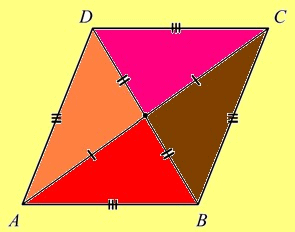Solution: Let ABCD be the given rhombus. Draw the diagonals AC and BD. Since AB || CDand AC is a transversal to AB and CD. We get
BAC = ∠ACD (alternate angles are equal) (1)
But AD CD (since ABCD is a rhombus)
∴∠ACD = ∠DAC
(angles opposite to the equal sides are equal)(2)
From (1) and (2), we get
BAC = ∠DAC
i.e., AC bisects the angle ∠A.
Similarly we can prove that AC bisects ∠CBD bisects ∠and BD bisects ∠D

Offer running on EduRev: Apply code STAYHOME200 to get INR 200 off on our premium plan EduRev Infinity!

,

,

,

,

,

,

,

,

,

,

,

,

,

,

,

,

,

,

,

,

,

,

,

,

,

,

,

,

,

,

;